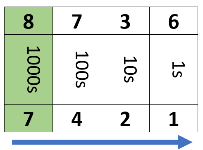# Math Worksheets Land

Math Worksheets For All Ages

# Math Worksheets Land

Math Worksheets For All Ages

# Comparing Large Numbers Worksheets

Being able to understand the relationship between two distinct values is an important component of being able to make sense of numbers and learning to evaluate data. We usually start this off by ordering numbers in a sequence from greatest to least or least to greatest. It all begins with understanding the significance of the basic integers 0 to 9. At this point we are entirely focused on whole numbers, but as we make our way into using decimals, fractions, and percentage this becomes much more critical. These worksheets and lessons show students how to compare numbers that are composed of a large number of digits.

### Aligned Standard: Grade 4 Base Ten - 4.NBT.2

• Answer Keys - These are for all the unlocked materials above.

### Homework Sheets

1. We give you integer, you give us the number name, expanded form, and place values. 2. Compare the underlined place value. 3. Name just the place value.

• Homework 1 - Write each number a) as a number name. b) in expanded form. c) as a number of hundreds, tens, and ones.
• Homework 2 - Compare the underlined values by writing the symbols >, <, or =.
• Homework 3 - Write the place value of the underlined digit.

### Practice Worksheets

Everything that you will find in the homework wrapped into one nicely neat package.

• Practice 1 - Convert the number that you are given to the form that is called for in each case.
• Practice 2 - Find the final value of the following problem.
• Practice 3 - It's all about place. You will convert a value to a number name, expanded form, and break it up in base ten units.

### Math Skill Quizzes

You might find yourself work hard on these.

• Quiz 1 - Very well spaced out questions for students.
• Quiz 2 - Convert a number of hundreds, tens, and ones.
• Quiz 3 - You can handle this in one quick swoop. Compare values and switch between expanded form and number names.

### How to Compare Large Numbers

When studying number systems, a comparison between two numbers is an important concept. A lot of kids struggle to determine which number is greater or lower in value. The concept of "greater-than" and "lesser-than" help in the basic addition and subtraction problems. When solving differences with subtraction, the position of the integers plays an essential role. When you subtract the lesser number from the greater number, you get a positive integer as the answer. When you subtract a greater number from a smaller number, you get a negative integer as the answer.

When ever you compare the value of two different values the best approach is focus on the place values of the integers within the number. When you must compare two multi-digit numbers which do not have an equal number of digits in it, things are very simple. You must count the number of digits, and the one with higher number of available place values in it is the greater number.

Example; which number is greater: 456 or 8,736? You can count the digits, 456 has three digits, while 8736 has four digits. Here is how you can identify the place values. The number 8736 has the highest place value of a thousand, while the highest place value in 456 is hundreds. It is an indication that the number 8736 is greater than the other number as it is in the thousands.

If the two numbers you are comparing have the equal number of digits, you need to carry out a comparison of the integers that are found within each of the place values.

Which of these values is largest: 8,736 or 7,421?

Start comparing from the highest place value. If the first digit of both numbers is different, you need to look for the bigger number. In this case, 8 is a greater number than 7, which makes 8,736 the bigger number. If the first digits of both these numbers were to be the same, you would have to continue the next place value, so on and so forth!

It will often help you to use the grid method for doing this. You create a grid with one value at the top and the place values written below them. Then you write the other number directly below it. An example of our last problem would look like this:As you can see, we compare the highest place value (1000s). If one integer is larger than the other, that is the largest number. If the integers are the same, we move one place to the right and compare those integers. We continue on with this until we find one integer that is larger than the other.

Unlock all the answers, worksheets, homework, tests and more!
Save Tons of Time! Make My Life Easier Now

## Thanks and Don't Forget To Tell Your Friends!

I would appreciate everyone letting me know if you find any errors. I'm getting a little older these days and my eyes are going. Please contact me, to let me know. I'll fix it ASAP.